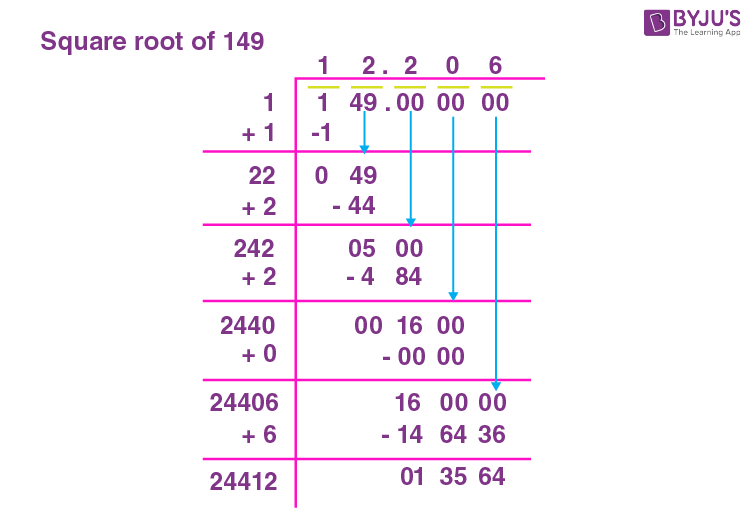# Square Root of 149

The square root of 149 is irrational. The approximate value of the square root of 149 is 12.2. When we say the square root of a number, that number is multiplied by itself to get a resultant number called the perfect square number. The number multiplied by itself is called the square root. Representing it with Mathematical notation would be “n × n = r”, where ‘r’ is the perfect square number and “n” is its square root. Note that “n” can be an integer. The methods to find “n” are either by prime factorisation method, long division method or repeated subtraction method. You can refer to the Finding Square root with Example.

Note the Following:

• The Square root of 149 = 2√149 = √149 where ‘√’ is the radical, and 149 is the radicand and 2 is the index.
• Exponential Form of Square root of 149 = 1491/2
• Solution for √149 ≈ 12.2
• The square root of 149 is Irrational = True

## What is the Square root of 149?

The square root of 149 is12.2, as the square root of 149 is irrational.

 √149 = irrational =12.2

## How to Find the Square root of 149?

There are three methods to find the Square root of 149

• Prime Factorisation method
• Long Division method
• Repeated Subtraction method

### Square root of 149 by Prime Factorisation Method

In the prime factorization method, 149 is divided by prime factors. Since 149 is a prime number, the only factors are 1 and 149. The square root of 149 cannot be further simplified with this method.

The division on 149 using prime factors is given below:

 149 149 × 1

### Square root of 149 by Long Division Method

To understand the long division method, follow the below-mentioned detailed steps;

Step 1: Group the given number into pairs.

Step 2: Divide the first pair, using the divisor such that the product is < or =.

Step 3: Continue the division by bringing the next pair

Step 4: The first divisor in the next division to be  (Divisor of first division + quotient of first division) such that;

d1 × 1 =

d2 × 2 =

d3 x 3 =

And so on.

Step 5: If the division is complete with the remainder as zero, then the quotient will be the square root. Else the number is not a perfect square number.

Applying the above steps to 149.

Pairing 1 and 49.

The squares are:

1 × 1 = 1

2 × 2 = 4Hence 149 does not have a perfect square. Therefore 149 is an irrational number whose root lies between 12 and 13.

### Square root of 149 by Repeated Subtraction Method.

When 149 is subtracted by one, and the resultant by 3 and it’s resultant by 5. Continuing the subtraction repeatedly by odd numbers is the repeated subtraction method of finding the square root. When this process results in zero at any step, then the step is the perfect square root of 149, else the number 149 is irrational.

The table below shows repeated subtraction for the given number 149.

 Step 1 149 – 1 = 148 Step 2 148 – 3 = 145 Step 3 145 – 5 = 140 Step 4 140 – 7 = 133 Step 5 133 – 9 = 124 Step 6 124 – 11 = 113 Step 7 113 – 13 = 100 Step 8 100 – 15 = 85 Step 9 85 – 17 = 68 Step 10 68 – 19 = 49 Step 11 49 – 21 = 28 Step 12 28 – 23 = 5 Step 13 5 – 25 = -20

Since the difference does not become zero, 149 is not a perfect square number and hence it does not have a perfect square root. The root lies between 12 and 13, resulting in a decimal root.

## Video Lessons

### Visualising square roots### Finding Square roots## Solved Examples

1. Does 149 have a perfect square root?

Solution: No. 149 does not have a perfect square root.

2. What is the square of 149?

Solution: 149 square is 22201.

## Frequently Asked Questions on Square root of 149

### What is the Square root of 149?

The Square root of 149 is 12.2.

### 149 is a perfect square number. True or False?

False. 149 is not a perfect square number.

### What are the factors of 149?

The factors of 149 are 1 and 149.

### Simplify √149?

√149 = √(1 × 149) no further simplification can be done.

### The square root of 149 is an irrational number. True or False?

True. The square root of 149 is an irrational number.